# GMAT Math : Profit

## Example Questions

### Example Question #1 : Dsq: Calculating Profit

An amusement park sells Children and Adult tickets. What was the total revenue for the day?

Statement 1: The amusement park sold 259 Children tickets and 345 Adult tickets.

Statement 2: Children tickets cost $32 and Adult tickets cost$45.

Statements 1 and 2 together are NOT sufficient.

EACH statement ALONE is sufficient.

Statement 2 ALONE is sufficient, but Statement 1 is not sufficient.

BOTH statements TOGETHER are sufficient, but NEITHER statement ALONE is sufficient.

Statement 1 ALONE is sufficient, but Statement 2 is not sufficient.

BOTH statements TOGETHER are sufficient, but NEITHER statement ALONE is sufficient.

Explanation:

Statement 1 gives us the number of tickets sold but not the price. Insufficient.

Statement 2 gives us the price of the tickets but not the number sold. Insufficient.

Together, the two statements give us both the number of tickets sold AND the price of each ticket. From this we can calculate the total revenue.

Note: We are only trying to determine if we have enough information to answer the question. We don't have to actually do the computations!

### Example Question #81 : Data Sufficiency Questions

How much money did Mary make this week?

Statement 1: Mary worked 40 hours of regular time and an additional 6 hours of overtime.

Statement 2: Mary made $30 an hour during normal working hours and$37 an hour during overtime.

EACH statement ALONE is sufficient.

Statements 1 and 2 TOGETHER are NOT sufficient.

BOTH statements TOGETHER are sufficient, but NEITHER statement ALONE is sufficient.

Statement 2 ALONE is sufficient, but statement 1 is not sufficient.

Statement 1 ALONE is sufficient, but statement 2 is not sufficient.

BOTH statements TOGETHER are sufficient, but NEITHER statement ALONE is sufficient.

Explanation:

We need both statements to find out how much money Mary made.

Statement 1 gives the type and number of hours, and statement 2 gives the amount she made per hour. Both together are sufficient, but neither is sufficient alone.

### Example Question #1 : Dsq: Calculating Profit

Jorge runs a business making picture frames.

I) Jorge made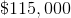in gross profit last year,more than the previous year.

II) Jorge had a profit margin of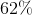.

What was Jorge's net profit?

Statement II is sufficient to answer the question, but statement I is not sufficient to answer the question.

Statement I is sufficient to answer the question, but statement II is not sufficient to answer the question.

Either statement alone is sufficient to answer the question.

Both statements are necessary to answer the question.

Both statements are necessary to answer the question.

Explanation:

Ignore the comment about 15% more than the previous year. We want to find net profit and in statement one we are given the gross profit. Statement II gives us the profit margin or percent profit.

We can use percent profit and gross profit to find net profit, but we cannot do it with only I or only II. Thus, they are both needed.

### Example Question #1 : Profit

An online store sells costum computers. Find the profit the store made on a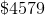sale.

I) The computer cost the store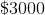to build.

II) The store generally makes a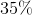profit.

Both statements are needed to answer the question.

Statement II is sufficient to answer the question, but statement I is not sufficient to answer the question.

Either statement is sufficient to answer the question.

Statement I is sufficient to answer the question, but statement II is not sufficient to answer the question.

Either statement is sufficient to answer the question.

Explanation:

To find the profit, we either need to know the cost or the percent profit.

I) Gives us the cost.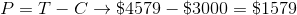II) Gives us the percent profit.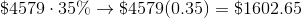Either of these can be used to find profit.

### All GMAT Math Resources